# How to Program the MAX14906 Quad-Channel Industrial Digital Output, Digital Input

### Abstract

The MAX14906 an IEC 61131-2 compliant, high-speed, four-channel industrial digital output, digital input device that can be configured on a per-channel basis as a high-side (HS) switch, a push-pull (PP) driver, or a Type 1 and 3, or Type 2 digital input. A microcontroller-compatible serial peripheral interface (SPI) provides access to many diagnostic features. This application note provides example C-code implementation including setup, monitoring, and diagnostic functions.

### Introduction

The MAX14906 is an IEC 61131-2 compliant, high-speed, four-channel industrial digital output, digital input device that can be configured on a per-channel basis as a high-side (HS) switch, a push-pull (PP) driver, or a Type 1 and 3, or Type 2 digital input. The SPI interface has a built-in chip addressing decoder, which allows communication with multiple MAX14906 devices utilizing a shared SPI with a common chip select (CS). The SPI interface provides flexibility for global and per-channel configuration and diagnostics, including supply overvoltage and undervoltage detection, wire-break or open-wire detection, thermal overload, and current limit reporting, and more.

This application note presents a series of functions to provide an easy and proven solution to program the MAX14906 (Figure 1). They are written in C# and should prove easy to port over to any common microcontroller. For detailed information about MAX14906 pins, operating modes, SPI commands and control registers, refer to the MAX14906 data sheet.### MAX14906 SPI

The MAX14906 has a high-speed SPI serial interface with maximum clock rate of 10MHz. The SPI interface adheres with clock polarity CPOL = 0 (SCLK idle = 0) and clock phase CPHA = 0 (rising/first edge samples the data). The commands are clocked in most significant bit (MSb) first.

The MAX14906 SPI supports addressable SPI which, allows direct communication with up to four MAX14906s using a shared CS signal. The address pins A1 and A0 are used to configure the device address. The address bits A1 and A0 are sent as the first and second bits of the SPI read and write command. The device monitors the SPI command and responds with SDO appropriately when the address matches the status of the A1 and A0 pins.

The MAX14906 SPI supports single-cycle mode and burst mode. The single-cycle mode reads or writes one register at a time, while burst mode allows reading or writing multiple consecutive registers in one SPI cycle.

The single-cycle SPI commands are 16 bits long (8-bit instruction + 8-bit data), with CRC disabled, and if CRC is enabled, this adds 8 more bits including 5-bit CRC and 3 leading zeros. The 2 MSBs of the command byte are device address bits (A1 and A0), which allow 4 MAX14906s to share the same chip select (CS) pin. The BRST bit is set to 0 for single-cycle mode. Data sent by the device on SDO reports the device fault conditions, as well as per-channel status if it is a write command or register value if it is a read command. Single-cycle read and write diagrams are shown from Figure 2 to Figure 5.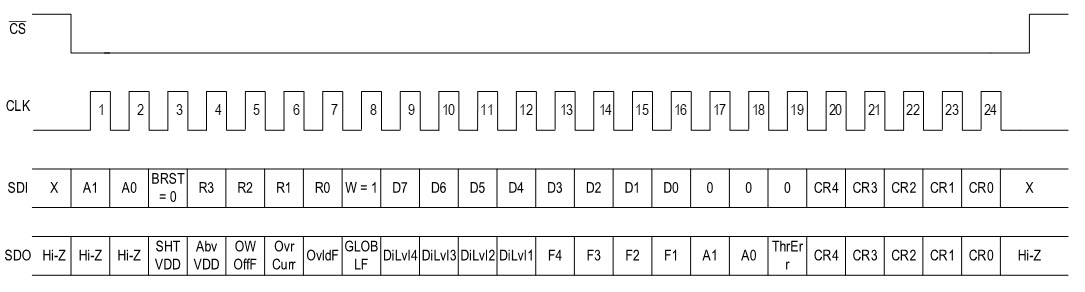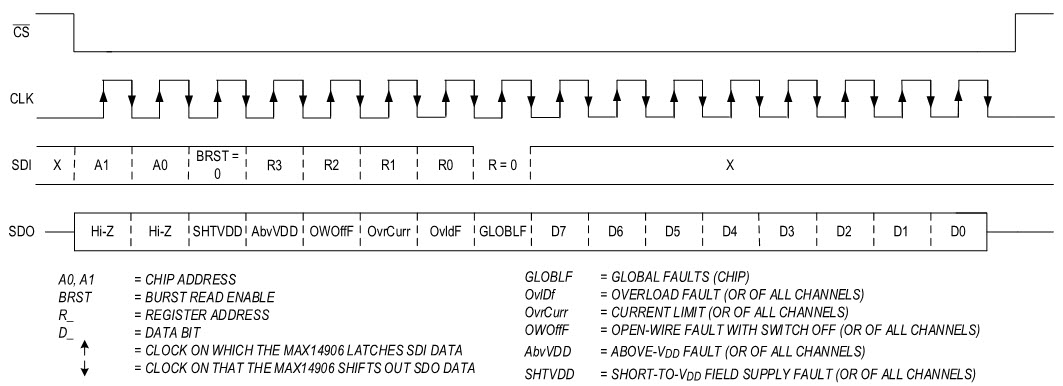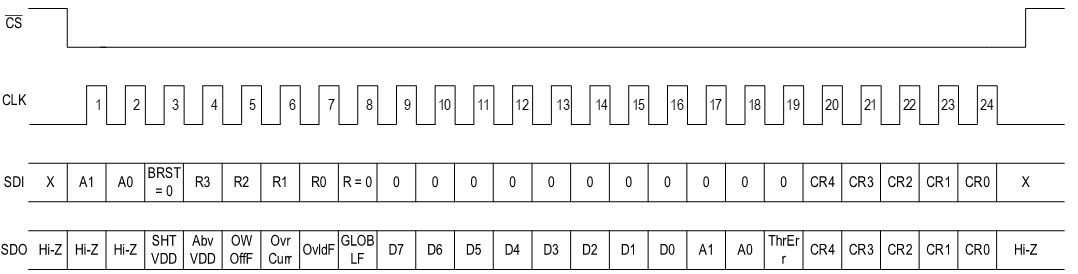The burst SPI command uses one SPI cycle and one register address to write to or read from multiple consecutive registers and is enabled by setting the BRST bit to 1.

The burst write command writes to both SetOUT and SetLED registers with BRST = 1, R/W = 1, and R[3:0] = 0. The command contains two data bytes, one to configure the SetOUT register and the other to configure the SetLED register. The number of SCLK cycles is 24 if CRC is not enabled and is 32 if CRC is enabled. SPI burst write diagrams are shown in Figure 6 and Figure 7.

The burst read command retrieves the data from six consecutive diagnostic registers from the address 0x02 to 0x07 (DoiLevel, Interrupt, OvrLdChF, OpnWirChF, ShtVDDChF, and GlobalErr registers) with BRST = 1, R/W = 0, R[3:0] = 2. The number of SCLK cycles is 56 if CRC is not enabled and is 64 if CRC is enabled. During a burst read, data bits 9 to 59 in the SDI data stream can be 0 or 1, but these bits are used to calculate the CRC bits if CRC is enabled. SPI burst read diagrams are shown in Figure 8 and Figure 9.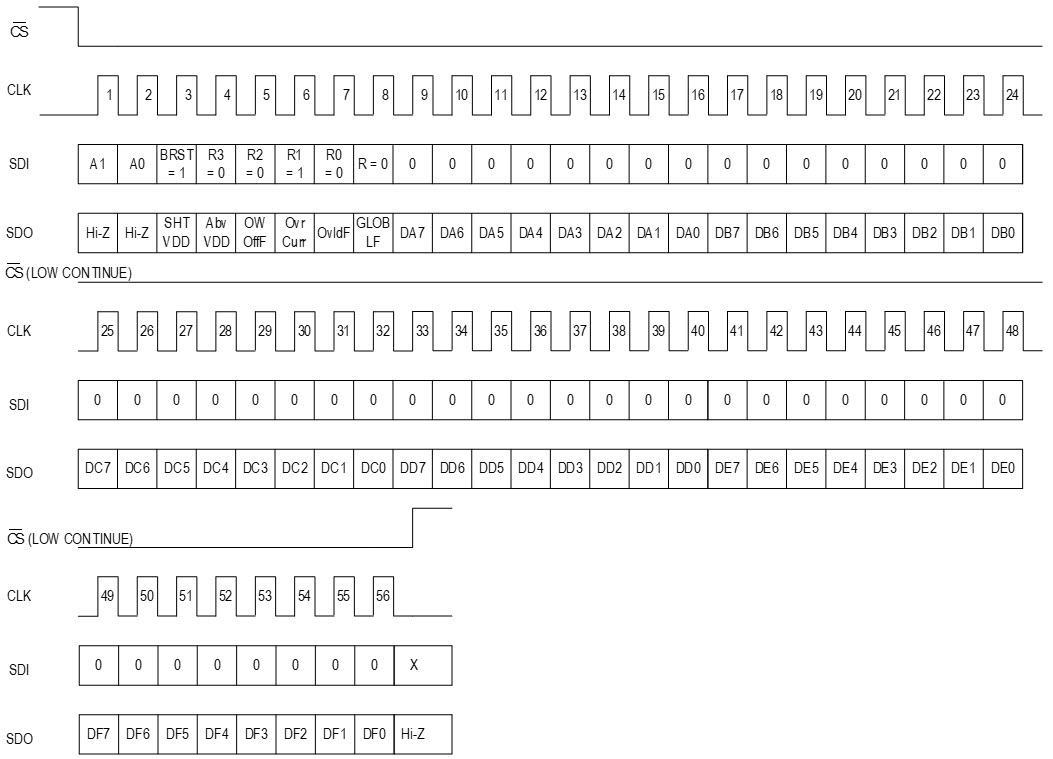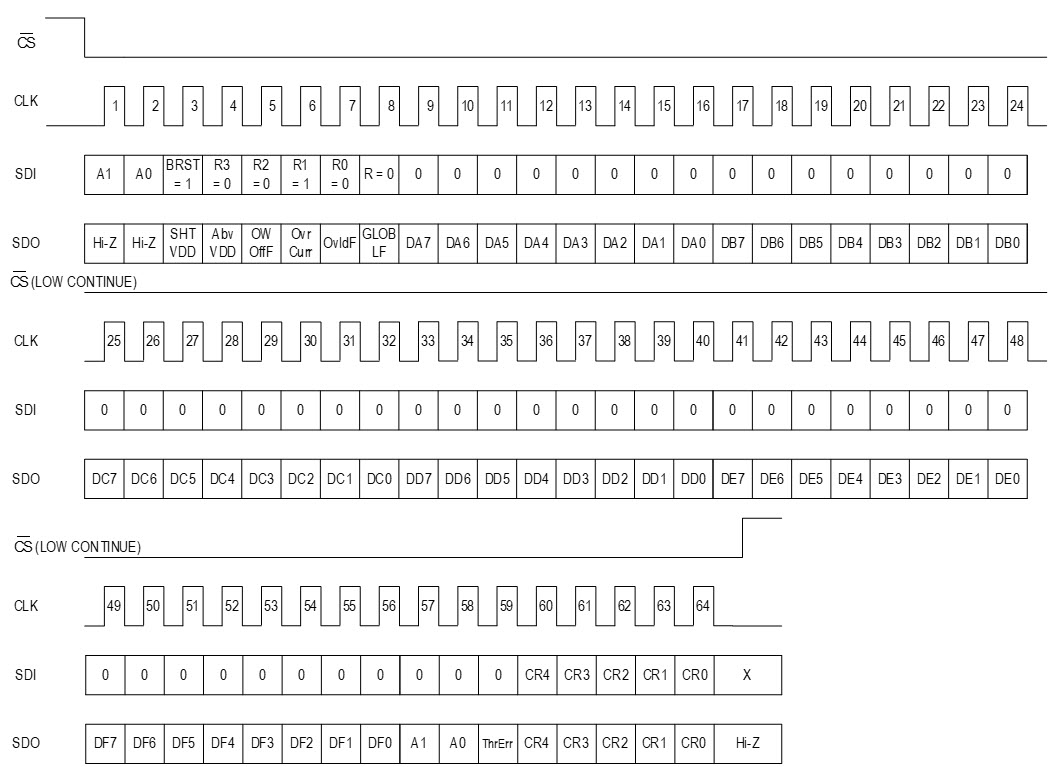For more details of SPI commands with the register tables and instructions, refer to the MAX14906 data sheet.

### MAX14906 - Code Application Examples

The MAX14906 is designed to support industrial applications in end equipment such as programmable logic controllers (PLCs) that require configurable digital input (Type 1/3 or Type 2) or digital output (high-side switch or push-pull driver). A typical application circuit that supports 4 channels group isolated, using a single MAX14483 digital isolator is shown in Figure 1.

### Source Code

This application note provides C# source code examples, essentially providing driver functions to access the multiple registers within the MAX14906 for configuration, control, and diagnostic features. All software has been implemented and tested using MAX14906 EV kit. The functions in this application note are used in the MAX14906 EV kit software to handle basic MAX14906 SPI read and write commands. It is designed to work with FT2232 microcontroller on the MAX14906 EV kit hardware. The USB communication and low-level FT2232 functions are not included in this document. The graphic interface functions are also not included in this document.

Customers should use the functions in this document as reference only and design their own firmware/software based on their own microcontroller and hardware implementation in their application.

### Single-Cycle Write

```            public void WriteRegister(int index)
{
if (index < 0 || index >= registers.Count) { return; }

byte register = 0x00;
byte[] txBuffer = new byte;
byte[] rxBuffer = new byte;

// Register Byte
register = 0x01;// Write
register |= (byte)(index << 1);// Register Address
txBuffer = register;
// Data Byte
txBuffer = registers[index].Value;

// Store Fault Data
SDOFaults.SHTVDD = ((rxBuffer & 0x20) >> 5);
SDOFaults.AbvVDD = ((rxBuffer & 0x10) >> 4);
SDOFaults.OWOffF = ((rxBuffer & 0x08) >> 3);
SDOFaults.OvrCurr = ((rxBuffer & 0x04) >> 2);
SDOFaults.OvldF = ((rxBuffer & 0x02) >> 1);
SDOFaults.GLOBLF = (rxBuffer & 0x01);
SDOFaults.ChannelFaults = rxBuffer;

// If successful, clean up
registers[index].Modified = false;
}
```

```        public void ReadRegister(int index)
{
if (index < 0 || index >= registers.Count) { return; }

FTDI.FT_STATUS ftStatus;
byte register = 0x00;
byte[] txBuffer = new byte;
byte[] rxBuffer = new byte;

// Register Byte
register |= (byte)(index << 1);// Register Address
txBuffer = register;
// Data Byte
txBuffer = 0x00;
ftStatus = SPIWriteRead(txBuffer, ref rxBuffer, 2);
if (ftStatus == FTDI.FT_STATUS.FT_OK)
{
registers[index].Value = rxBuffer;
}

// Store Fault Data
SDOFaults.SHTVDD = ((rxBuffer & 0x20) >> 5);
SDOFaults.AbvVDD = ((rxBuffer & 0x10) >> 4);
SDOFaults.OWOffF = ((rxBuffer & 0x08) >> 3);
SDOFaults.OvrCurr = ((rxBuffer & 0x04) >> 2);
SDOFaults.OvldF = ((rxBuffer & 0x02) >> 1);
SDOFaults.GLOBLF = (rxBuffer & 0x01);
//SDOFaults.ChannelFaults = rxBuffer;// Not Valid, bc on read this is register data

// If successful, clean up
registers[index].Modified = false;
}
```

### Burst Write

```        public void BurstWriteRegisters(int startIndex, int endIndex)
{
// Typically always 0 and 1 only
if (endIndex < startIndex) { return; }
if (startIndex < 0) { return; }
if (endIndex >= registers.Count) { return; }

int byteCount = (endIndex - startIndex) + 2;
byte register = 0x00;
byte[] txBuffer = new byte[byteCount];
byte[] rxBuffer = new byte[byteCount];

// Register Byte
register = 0x01;// Write
register |= (byte)(startIndex << 1);// Register Address
register |= (byte)(0x01 << 5);// Burst
txBuffer = register;

// Data Byte
for (int x = 0; x < (byteCount - 1); x++)
{
txBuffer[1 + x] = registers[startIndex + x].Value;
registers[startIndex + x].Modified = false;
}

// Store Fault Data
SDOFaults.SHTVDD = ((rxBuffer & 0x20) >> 5);
SDOFaults.AbvVDD = ((rxBuffer & 0x10) >> 4);
SDOFaults.OWOffF = ((rxBuffer & 0x08) >> 3);
SDOFaults.OvrCurr = ((rxBuffer & 0x04) >> 2);
SDOFaults.OvldF = ((rxBuffer & 0x02) >> 1);
SDOFaults.GLOBLF = (rxBuffer & 0x01);
SDOFaults.ChannelFaults = rxBuffer;
}

```

```        public void BurstReadRegisters(int startIndex, int endIndex)
{
if (endIndex < startIndex) { return; }
if (startIndex < 0) { return; }
if (endIndex >= registers.Count) { return; }

FTDI.FT_STATUS ftStatus;
int byteCount = (endIndex - startIndex) + 2;
byte register = 0x00;
byte[] txBuffer = new byte[byteCount];
byte[] rxBuffer = new byte[byteCount];

// Register Byte
register |= (byte)(startIndex << 1);// Register Address
register |= (byte)(0x01 << 5);// Burst
txBuffer = register;

// Data Byte
for (int x = 0; x < (byteCount - 1); x++)
{
txBuffer[1 + x] = 0x00;
}
ftStatus = SPIWriteRead(txBuffer, ref rxBuffer, byteCount);

if (ftStatus == FTDI.FT_STATUS.FT_OK)
{
for (int x = 0; x < (byteCount - 1); x++)
{
registers[startIndex + x].Value = rxBuffer[1 + x];
registers[startIndex + x].Modified = false;
}
}

// Store Fault Data
SDOFaults.SHTVDD = ((rxBuffer & 0x20) >> 5);
SDOFaults.AbvVDD = ((rxBuffer & 0x10) >> 4);
SDOFaults.OWOffF = ((rxBuffer & 0x08) >> 3);
SDOFaults.OvrCurr = ((rxBuffer & 0x04) >> 2);
SDOFaults.OvldF = ((rxBuffer & 0x02) >> 1);
SDOFaults.GLOBLF = (rxBuffer & 0x01);
}
```

### CRC Calculation

```    private CRC8 crc8 = new CRC8(0x15, 0x1F);

class CRC8
{
private readonly byte POLY = 0x00;
private readonly byte START_VALUE = 0x00;

public CRC8(byte poly, byte startValue = 0x00)
{
this.POLY = poly;
this.START_VALUE = startValue;
}

public byte ComputeChecksum3MSB(byte[] bytes)
{
byte remainder = START_VALUE;
byte min = 0;
for (int bite = 0; bite < bytes.Length; ++bite)
{
// For MAX14906 it does 8/16bits plus the 3 "0" MSBs of check byte
if (bite == (bytes.Length - 1)) { min = 5; }

for (byte bit = 8; bit > min; --bit)
{
remainder = (((bytes[bite] >> (bit - 1) & 0x01) ^ ((remainder >> 4) & 0x01)) > 0) ? (byte)((remainder << 1) ^ POLY) : (byte)(remainder << 1);
}
}
return (byte)(remainder & 0x1F);
}
}
```

### Example to Configure Operation Mode for Each Channel

```        public void SendModeAndSetting(int channel, int mode, int setting)
{
if (channel < 1 || channel > 4) { return; }
if (mode < 0 || mode > 2) { return; }
if (((mode == 1) && (setting < 0 || setting > 4)) || ((mode == 0) && (setting < 0 || setting > 2)) || (mode == 2 && setting != 0)) { return; }

byte register = 0x00;
byte data = 0x00;
byte[] txBuffer = new byte;
byte[] rxBuffer = new byte;

if (mode == 0 || mode == 2)// Input or Low-leakage
{
////////////////////////////
// Reg 0x00:
///////////////////////////
// Register Byte
register = 0x01;// Write
register |= (0x00 << 1);// Register Address
txBuffer = register;
// Data Byte
// Set current bit mode from FTDI
byte temp = 0x00;
// Remember bit logic for FTDI input/output inverted from MAX14906 Reg 0x00
temp = (byte)((~FTDI_ADBUS_MODE & 0x80) >> 7);// DIO1
data |= (byte)(temp << 4);
temp = (byte)((~FTDI_ACBUS_MODE & 0x01));// DIO2
data |= (byte)(temp << 5);
temp = (byte)((~FTDI_ACBUS_MODE & 0x02) >> 1);// DIO3
data |= (byte)(temp << 6);
temp = (byte)((~FTDI_ACBUS_MODE & 0x04) >> 2);// DIO4
data |= (byte)(temp << 7);
// New Mode to Set
temp = (byte)(0x01 << (channel + 3));
data = (mode == 0 || mode == 2) ? (byte)(temp | data) : (byte)(~temp & data);
txBuffer = data;

////////////////////////////
// Reg 0x0C:
///////////////////////////
// Register Byte
register |= (0x0C << 1);// Register Address
txBuffer = register;
// Get Current Data from Reg 0x0C
temp = rxBuffer;

// Register Byte
register = 0x01;// Write
register |= (0x0C << 1);// Register Address
txBuffer = register;
// Data Byte
data = (setting == 1) ? (byte)(temp | 0x80) : (byte)(temp & ~0x80);// Type 2 IEC Mode, Note this forces all DI to be in this mode
txBuffer = data;

////////////////////////////
// Reg 0x0D:
///////////////////////////
// Register Byte
register = 0x01;// Write
register |= (0x0D << 1);// Register Address
txBuffer = register;
// Data Byte
data = (byte)(DO_Settings & ~(0x03 << (channel - 1) * 2));// Whether any setting (Normal, IEC 2, or Low-leakage) zero out both bits
if (mode == 2)// Low-leakage
{
data |= (byte)(0x02 << (channel - 1) * 2);// For Low-leakage DI must set PP
}
DO_Settings = data;

txBuffer = data;
}
else// Output
{
////////////////////////////
// Reg 0x00:
///////////////////////////
// Register Byte
register = 0x01;// Write
register |= (0x00 << 1);// Register Address
txBuffer = register;
// Data Byte
// Set current bit mode from FTDI
byte temp = 0x00;
// Remember bit logic for FTDI input/output inverted from MAX14906 Reg 0x00
temp = (byte)((~FTDI_ADBUS_MODE & 0x80) >> 7);// DIO1
data |= (byte)(temp << 4);
temp = (byte)((~FTDI_ACBUS_MODE & 0x01));// DIO2
data |= (byte)(temp << 5);
temp = (byte)((~FTDI_ACBUS_MODE & 0x02) >> 1);// DIO3
data |= (byte)(temp << 6);
temp = (byte)((~FTDI_ACBUS_MODE & 0x04) >> 2);// DIO4
data |= (byte)(temp << 7);
// New Mode to Set
temp = (byte)(0x01 << (channel + 3));
data = (mode == 0) ? (byte)(temp | data) : (byte)(~temp & data);
txBuffer = data;

////////////////////////////
// Reg 0x0D:
///////////////////////////
// Register Byte
register = 0x01;// Write
register |= (0x0D << 1);// Register Address
txBuffer = register;
// Data Byte
data = (byte)(DO_Settings & ~(0x03 << (channel - 1) * 2));// Whether any setting (HS, HS 2x, PP clamp, PP low) zero out both bits
data |= (byte)(setting << (channel - 1) * 2);

DO_Settings = data;// Update settings

txBuffer = data;
}

// Store Fault Data
SDOFaults.SHTVDD = ((rxBuffer & 0x20) >> 5);
SDOFaults.AbvVDD = ((rxBuffer & 0x10) >> 4);
SDOFaults.OWOffF = ((rxBuffer & 0x08) >> 3);
SDOFaults.OvrCurr = ((rxBuffer & 0x04) >> 2);
SDOFaults.OvldF = ((rxBuffer & 0x02) >> 1);
SDOFaults.GLOBLF = (rxBuffer & 0x01);
SDOFaults.ChannelFaults = rxBuffer;
}
```

### Conclusion

This application note shows how to program the MAX14906 to monitor inputs, drive outputs, and diagnose fault-conditions. This code is tested by using MAX14906EVKIT#. An engineer can implement an interface quickly and easily between popular microcontrollers and the MAX14906 by using C-code examples mentioned in this application note.